UIHistories Project: A History of the University of Illinois by Kalev Leetaru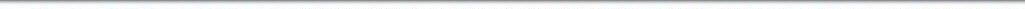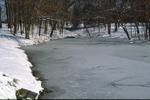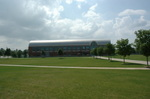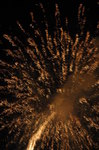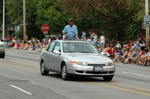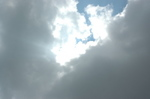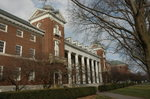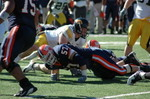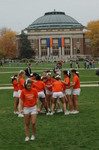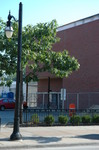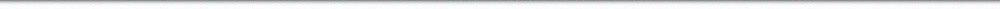N A V I G A T I O N D I G I T A L L I B R A R Y

## Repository: UIHistories Project: Course Catalog - 1876-1877 [PAGE 35]

Caption: Course Catalog - 1876-1877
This is a reduced-resolution page image for fast online browsing.

< Previous Page [Displaying Page 35 of 73] Next Page >
[VIEW ALL PAGE THUMBNAILS]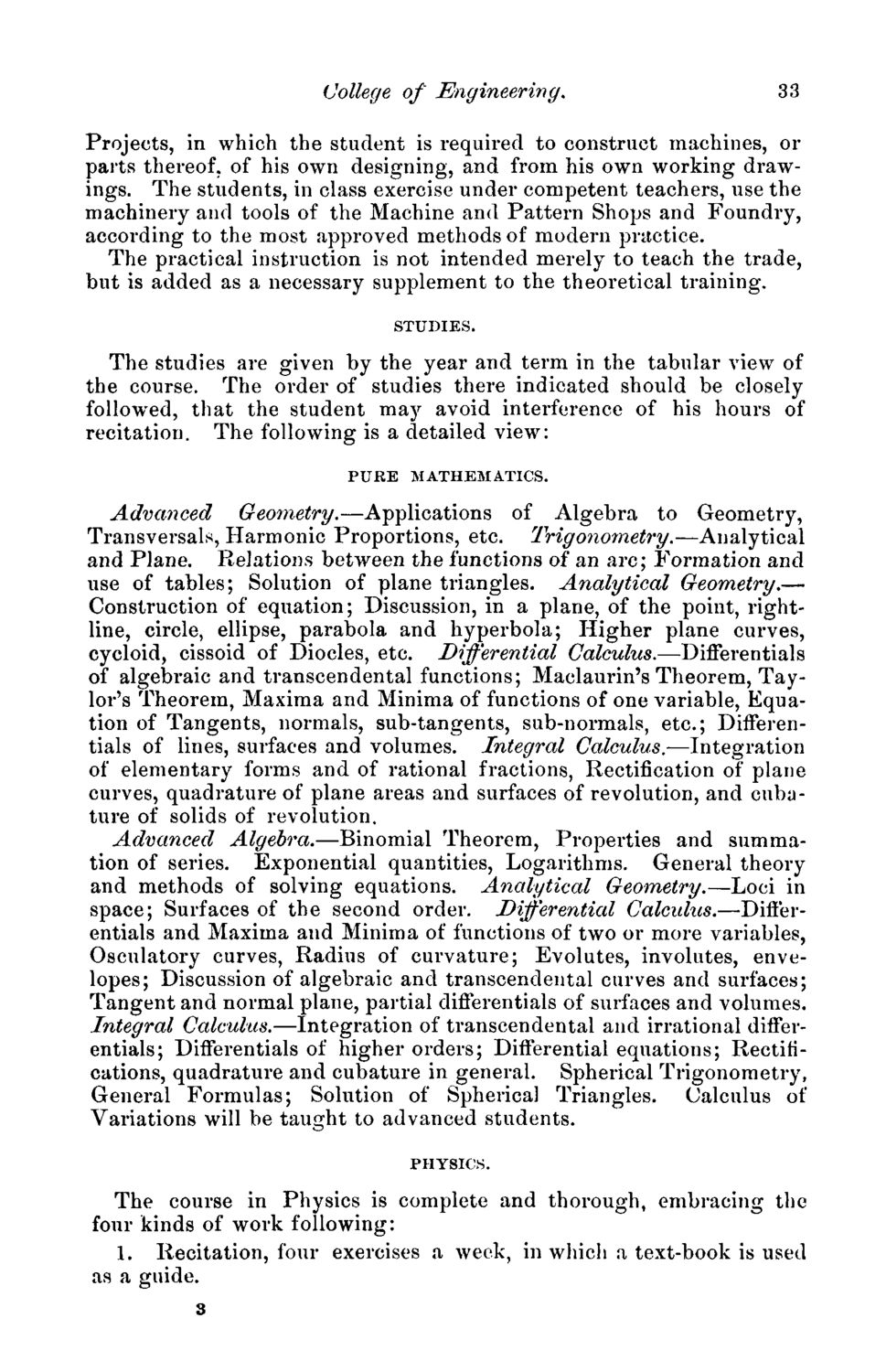### EXTRACTED TEXT FROM PAGE:

College of Engineering.

33

Projects, in which the student is required to construct machines, or parts thereof, of his own designing, and from his own working drawings. The students, in class exercise under competent teachers, use the machinery and tools of the Machine and Pattern Shops and Foundry, according to the most approved methods of modern practice. The practical instruction is not intended merely to teach the trade, but is added as a necessary supplement to the theoretical training.

STUDIES.

The studies are given by the year and term in the tabular view of the course. The order of studies there indicated should be closely followed, that the student may avoid interference of his hours of recitation. The following is a detailed view:

PURE MATHEMATICS.

Advanced Geometry.—Applications of Algebra to Geometry, Transversals, Harmonic Proportions, etc. Trigonometry.—Analytical and Plane. Relations between the functions of an arc; Formation and use of tables; Solution of plane triangles. Analytical Geometry.— Construction of equation; Discussion, in a plane, of the point, rightline, circle, ellipse, parabola and hyperbola; Higher plane curves, cycloid, cissoid of Diocles, etc. Differential Calculus.—Differentials of algebraic and transcendental functions; Maclaurin's Theorem, Taylor's Theorem, Maxima and Minima of functions of one variable, Equation of Tangents, normals, sub-tangents, sub-normals, etc.; Differentials of lines, surfaces and volumes. Integral Calculus.—Integration of elementary forms and of rational fractions, Rectification of plane curves, quadrature of plane areas and surfaces of revolution, and cubyture of solids of revolution. Advanced Algebra.—Binomial Theorem, Properties and summation of series. Exponential quantities, Logarithms. General theory and methods of solving equations. Analytical Geometry.—Loci in space; Surfaces of the second order. Differential Calculus.—Differentials and Maxima and Minima of functions of two or more variables, Oscillatory curves, Radius of curvature; Evolutes, involutes, envelopes; Discussion of algebraic and transcendental curves and surfaces; Tangent and normal plane, partial differentials of surfaces and volumes. Integral Calculus.—Integration of transcendental and irrational differentials; Differentials of higher orders; Differential equations; Rectifications, quadrature and cubature in general. Spherical Trigonometry, General Formulas; Solution of Spherical Triangles. Calculus of Variations will be taught to advanced students.

PHYSICS.

The course in Physics is complete and thorough, embracing the four kinds of work following: 1. Recitation, four exercises a week, in which a text-book is used as a guide.

3